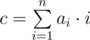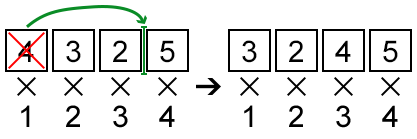# CF631E Product Sum

• 47通过
• 190提交
• 题目来源
• 评测方式 RemoteJudge
• 标签
• 难度 NOI/NOI+/CTSC
• 时空限制 1000ms / 256MB
• 提示：收藏到任务计划后，可在首页查看。

## 题意翻译

有一个长为 $n$ 的序列 $A_{i}$​，定义这个序列的价值为$\sum_{i=1}^n i*A_{i}$。

现在对这个序列进行一次操作： 将序列中的某个数移到序列中的某一个位置，例如 $1,2,3,4$ 将 $4$ 移到 $1$ 位置上，序列就变成了 $4,1,2,3$。

现要进行一次操作，使得这个序列的价值最大

输入格式：

两行。第一行一个整数 $n$，表示这个序列长度；第二行 $n$ 个整数，表示序列 $A$

输出格式：

一个整数，表示这个序列的最大价值

说明： $2 \leq n \leq 2*10^{5}$ $|A_{i}| \leq 10^{6}$

## 题目描述

Blake is the boss of Kris, however, this doesn't spoil their friendship. They often gather at the bar to talk about intriguing problems about maximising some values. This time the problem is really special.

You are given an array $a$ of length $n$ . The characteristic of this array is the value— the sum of the products of the values $a_{i}$ by $i$ . One may perform the following operation exactly once: pick some element of the array and move to any position. In particular, it's allowed to move the element to the beginning or to the end of the array. Also, it's allowed to put it back to the initial position. The goal is to get the array with the maximum possible value of characteristic.## 输入输出格式

输入格式：

The first line of the input contains a single integer $n$ ( $2<=n<=200000$ ) — the size of the array $a$ .

The second line contains $n$ integers $a_{i}$ ( $1<=i<=n$ , $|a_{i}|<=1000000$ ) — the elements of the array $a$ .

输出格式：

Print a single integer — the maximum possible value of characteristic of $a$ that can be obtained by performing no more than one move.

## 输入输出样例

输入样例#1： 复制
4
4 3 2 5

输出样例#1： 复制
39
输入样例#2： 复制
5
1 1 2 7 1

输出样例#2： 复制
49
输入样例#3： 复制
3
1 1 2

输出样例#3： 复制
9

## 说明

In the first sample, one may pick the first element and place it before the third (before $5$ ). Thus, the answer will be $3·1+2·2+4·3+5·4=39$ .

In the second sample, one may pick the fifth element of the array and place it before the third. The answer will be $1·1+1·2+1·3+2·4+7·5=49$ .

提示
标程仅供做题后或实在无思路时参考。
请自觉、自律地使用该功能并请对自己的学习负责。
如果发现恶意抄袭标程，将按照I类违反进行处理。